# Refresher Course on Matrix Analysis and Optimization

## Part II: Numerical Optimization

Franck Iutzeler Jerome Malick

We consider two $\mathbb{R}^2 \to \mathbb{R}$ convex functions with the same global minimizer $(3,1)$ but quite different shapes and see how this impacts the performance of gradient-based algorithms. The considered functions $f$ and $g$ and their 3D are:

\begin{array}{rrcll} f: & \mathbb{R}^2 & \to &\mathbb{R}\\ & (x_1,x_2) & \mapsto & 4 (x_1-3)^2 + 2(x_2-1)^2 \end{array} \begin{array}{rrcll} g: & \mathbb{R}^2 & \to &\mathbb{R}\\ & (x_1,x_2) & \mapsto & \log( 1 + \exp(4 (x_1-3)^2 ) + \exp( 2(x_2-1)^2 ) ) - \log(3) . \end{array}In numerical optimization, it is customary to define oracles for the functions. These are functions that take a point of the ambiant space as an input and return the value of the function at this point (or its gradient, Hessian, etc.)

In :
# Oracle for function f
def f(x):
"""
Function f
"""
x1 = x
x2 = x
return 4*(x1-3)**2+2*(x2-1)**2


Plotting the level sets of the functions can be useful in 2D.

In :
import numpy as np
import matplotlib.pyplot as plt

npoints = 200
xmin = -0.5
xmax = 5.5
ymin = -0.5
ymax = 5.5

In :
X,Y = np.meshgrid(np.linspace(xmin,xmax,npoints),np.linspace(ymin,ymax,npoints))

Points = [  np.array([x,y]) for (x,y) in zip(X.ravel(), Y.ravel())]

Z = np.array([ f(p) for p in Points ]).reshape(X.shape)

In :
plt.contour(X,Y,Z,levels=[0.1,1,2,5,10,25])

Out:
<matplotlib.contour.QuadContourSet at 0x7ff491ec75b0>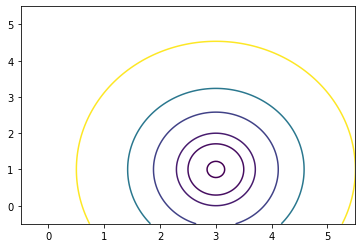Question a: Construct a function g that will be the oracle for the second function \begin{array}{rrcll} g: & \mathbb{R}^2 & \to &\mathbb{R}\\ & (x_1,x_2) & \mapsto & \log( 1 + \exp(4 (x_1-3)^2 ) + \exp( 2(x_2-1)^2 ) ) - \log(3) . \end{array}

In :
def g(x):
"""
Function g
"""
x1 = x
x2 = x
return np.log( 1 + np.exp(4*(x1-3)**2) + np.exp(2*(x2-1)**2) ) - np.log(3)


Question b: Create a function f_grad that returns $\nabla f(x)$ (as a Numpy array) from an input vector $x$. Do the same for function $g$

In :
def f_grad(x):
x1 = x
x2 = x
gx = 8*(x1-3)
gy = 4*(x2-1)
return np.array( [ gx  ,  gy  ] )

In :
def g_grad(x):
x1 = x
x2 = x
gx = 8*(x1-3)*np.exp(4*(x1-3)**2)/( 1 + np.exp(4*(x1-3)**2) + np.exp(2*(x2-1)**2) )
gy = 4*(x2-1)*np.exp(2*(x2-1)**2)/( 1 + np.exp(4*(x1-3)**2) + np.exp(2*(x2-1)**2) )
return np.array( [ gx  ,  gy  ] )


Question c: Implement a constant stepsize gradient method gradient_algorithm(f,f_grad,x0,step,PREC,ITE_MAX) that takes:

• f and f_grad: respectively functions and gradient oracles
• x0: a starting point
• step: a (real) stepsize
• PREC and ITE_MAX: two stopping criteria for i) sought precision (in gradient norm); and ii) maximum number of iterations;

and returns a tuple with x the final point and x_tab the matrix of all vectors stacked vertically

In :
import numpy as np
import timeit

def gradient_algorithm(f , f_grad , x0 , step , PREC , ITE_MAX ):
x = np.copy(x0)

x_tab = np.copy(x)
print("------------------------------------\n Constant Stepsize gradient\n------------------------------------\nSTART    -- stepsize = {:0}".format(step))
t_s =  timeit.default_timer()
for k in range(ITE_MAX):
x = x - step*g

x_tab = np.vstack((x_tab,x))

if np.linalg.norm(g) < stop:
break

t_e =  timeit.default_timer()
print("FINISHED -- {:d} iterations / {:.6f}s -- final value: {:f} at point ({:.2f},{:.2f})\n\n".format(k,t_e-t_s,f(x),x,x))
return x,x_tab


Question d: Test your gradient descent function on $f$ and $g$:

1. Verify that the final point is close to the sought minimizer $(3,1)$
• Observe the behavior of the iterates (you can plot them on top of the contour plot)
• Change the stepsize and give the values for which the algorithm i) diverges; and ii) oscillates. Compare with theoretical limits by computing the Lipschitz constant of the gradients
In :
def level_points_plot(f, x_tab, xmin, xmax, ymin, ymax, npoints, levels):
X,Y = np.meshgrid(np.linspace(xmin,xmax,npoints),np.linspace(ymin,ymax,npoints))

Points = [  np.array([x,y]) for (x,y) in zip(X.ravel(), Y.ravel())]
Z = np.array([ f(p) for p in Points ]).reshape(X.shape)
graph = plt.contour(X,Y,Z,levels=[0.1,1,2,5,10,25])

if x_tab.shape > 40:
sub = int(x_tab.shape/40.0)
x_tab = x_tab[::sub]

delay = 2.0/x_tab.shape
for k in range(x_tab.shape):
plt.plot(x_tab[k,0], x_tab[k,1], '*b', markersize=10)
plt.show()

In :
PREC    = 1e-8
ITE_MAX = 100
x0 = np.array([0, 0])
step = 0.1

npoints = 200
xmin = -0.5
xmax = 5.5
ymin = -1
ymax = 5.5
levels = [0.1,1,2,5,10,25]

level_points_plot(f, x_tab_f, xmin, xmax, ymin, ymax, npoints, levels)

------------------------------------
------------------------------------
START    -- stepsize = 0.1
FINISHED -- 33 iterations / 0.002031s -- final value: 0.000000 at point (3.00,1.00)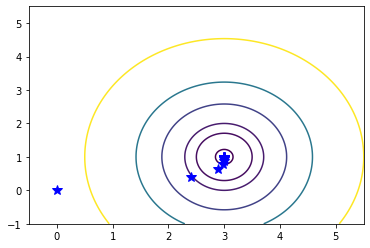In :
PREC    = 1e-8
ITE_MAX = 100
x0 = np.array([0, 0])
step = 0.1

npoints = 200
xmin = -0.5
xmax = 5.5
ymin = -1
ymax = 5.5
levels = [0.1,1,2,5,10,25]

level_points_plot(g, x_tab, xmin, xmax, ymin, ymax, npoints, levels)

------------------------------------
------------------------------------
START    -- stepsize = 0.1
FINISHED -- 99 iterations / 0.008473s -- final value: 0.000000 at point (3.00,1.00)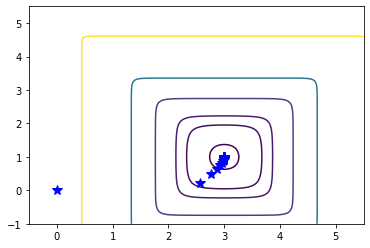# 2- Application to Regression¶

We now get back to the problem of predicting the final grade of a student from various features treated in the Matrix part of the course.

We remind that mathematically, from the $m_{learn} \times (n+1)$ learning matrix $A_{learn}$ ($m_{learn} = 300$, $n=27$) comprising of the features values of each training student in line, and the vector of the values of the target features $b_{learn}$; we seek a size-$(n+1)$ regression vector that minimizes the squared error between $A_{learn} x$ and $b_{learn}$. This problem boils down to the following least square problem: $$\min_{x\in\mathbb{R}^{n+1}} s(x) = \frac{1}{2} \| A_{learn} x - b_{learn} \|_2^2 .$$

In :
import numpy as np

A_learn = dat_file['A_learn']
b_learn = dat_file['b_learn']
A_test = dat_file['A_test']
b_test = dat_file['b_test']

m = 395 # number of read examples (total:395)
n = 27 # features
m_learn = 300


Question a: Construct the oracles for function $s$ and its gradient as in the previous section

In :
def s(x):
return 0.5 * np.linalg.norm(np.dot(A_learn, x) - b_learn)**2

return np.dot(A_learn.T, (np.dot(A_learn, x) - b_learn))


Question b: Compute the Lipschitz constant of the gradient of $s$. Find a solution to the minimization of $s$ using your gradient algorithm. Compare with Numpy's Least Square routine.

In :
L = np.linalg.norm(np.dot(A_learn.T, A_learn))
print(L)

PREC    = 1e-12
ITE_MAX = 5000
x0 = np.zeros(n+1)
step = 1 / L

x_lstsq = np.linalg.lstsq(A_learn, b_learn,rcond=None)

print(np.linalg.norm(x - x_lstsq))

1917.0184554201248
------------------------------------
------------------------------------
START    -- stepsize = 0.0005216433869859874
FINISHED -- 1090 iterations / 0.047718s -- final value: 373.404016 at point (0.02,0.02)

9.296353339218272e-11

In :
ys = [ np.linalg.norm(x_tab[k, :] - x_lstsq) for k in range(x_tab.shape)]

fig, ax = plt.subplots()
ax.semilogy(range(x_tab.shape), ys)
ax.set(xlabel='iteration k', ylabel='||x_k - x_lstsq ||')

Out:
[Text(0.5, 0, 'iteration k'), Text(0, 0.5, '||x_k - x_lstsq ||')]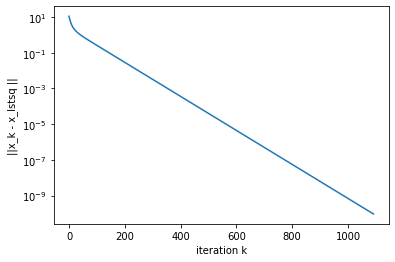Question c: Generate random Gaussian matrix/vector couples $A,b$ with different sizes, change the shape of $A$ from tall (nb. of rows >> nb. of cols.) to fat (nb. of rows << nb. of cols.). Compare the execution time of constant stepsize gradient with fixed precision for the different couples.

In :
import numpy as np

n_new = 100
m_new = 10000

A_learn = np.random.randn(m_new,n_new)
b_learn = np.random.randn(m_new)

PREC    = 1e-12
ITE_MAX = 5000
x0 = np.zeros(n_new)
step = 1 / np.linalg.norm(np.dot(A_learn.T, A_learn))

def s(x):
return 0.5 * np.linalg.norm(np.dot(A_learn, x) - b_learn)**2

return np.dot(A_learn.T, (np.dot(A_learn, x) - b_learn))


------------------------------------
------------------------------------
START    -- stepsize = 9.942919493341326e-06
FINISHED -- 301 iterations / 0.178198s -- final value: 4945.577981 at point (-0.02,-0.00)



# 3- Beyond constant stepsizes¶

The Rosenbrock function $r$ writes

\begin{array}{rrcll} r: & \mathbb{R}^2 & \to &\mathbb{R}\\ & (x_1,x_2) & \mapsto & (1-x_1)^2 + 100(x_2-x_1^2)^2 . \end{array}This function has a global minimum at $(1,1)$ with value $0$.

Question a: Construct function and gradient oracles for $r$.

In :
def r(x):
x1 = x
x2 = x
return (1-x1)**2 + 100*(x2-x1**2)**2

x1 = x
x2 = x
g1 = 2*(x1-1) + 100*2*(2*x1)*(x1**2-x2)
g2 = 100*2*(x2-x1**2)
return np.array([g1,g2])


Question b: Try to minimize $r$ using your constant stepsize gradient function. Can you find a stepsize for which the algorithm converges?

In :
PREC    = 1e-6
ITE_MAX = 1000
x0 = np.zeros(2)
step = 0.001


------------------------------------
------------------------------------
START    -- stepsize = 0.001
FINISHED -- 999 iterations / 0.038355s -- final value: 0.106595 at point (0.67,0.45)


In :
npoints = 200
xmin = -1.5
xmax = 2.5
ymin = -1.5
ymax = 2.5
levels = [0.1,1,2,5,10,25]

level_points_plot(r, x_tab, xmin, xmax, ymin, ymax, npoints, levels)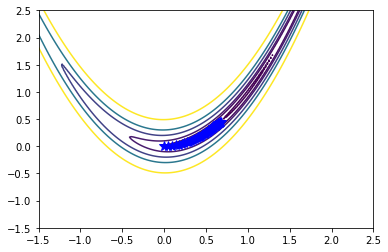The observed problem is typical of poorly conditionned functions. At the beggining, gradients are large and so a small stepsize has to be employed but near the optimal value, the gradients are very small and thus the progress is very slow.

Question c: Try to devise a gradient method where you change the stepsize during the algorithms to mend this problem.

In [ ]:



A systematic approch to stepsize tuning is the use of a line-search method.

Question d: Implement a gradient algorithm with Armijo-Wolfe linesearch using Scipy's line_search routine.

In :
import numpy as np
import timeit
from scipy.optimize import line_search

x = np.copy(x0)
stop = PREC*np.linalg.norm( g )

x_tab = np.copy(x)
print("------------------------------------\n Gradient with Wolfe line search\n------------------------------------\nSTART")
t_s =  timeit.default_timer()
for k in range(ITE_MAX):

########### TO FILL
stepsize = line_search(f, f_grad, x, -g)
x = x - stepsize*g  ###### ITERATION

x_tab = np.vstack((x_tab,x))

if np.linalg.norm(g) < stop:
break
t_e =  timeit.default_timer()
print("FINISHED -- {:d} iterations / {:.6f}s -- final value: {:f} at point ({:.2f},{:.2f})\n\n".format(k,t_e-t_s,f(x),x,x))
return x,x_tab

In :
x, x_tab = gradient_algorithm(r, r_grad, x0, step, PREC, ITE_MAX)

------------------------------------
------------------------------------
START    -- stepsize = 0.001
FINISHED -- 999 iterations / 0.046015s -- final value: 0.106595 at point (0.67,0.45)


In :
x, x_tab = gradient_Wolfe(r, r_grad, x0, PREC, ITE_MAX)

------------------------------------
------------------------------------
START
FINISHED -- 999 iterations / 0.395643s -- final value: 0.000176 at point (0.99,0.97)



# 4- Application to Classification¶

Binary classification is another popular problem in machine learning. Instead of predicting a numerical value, the goal is now to classify the student into two classes: $+1$ -- pass i.e. final grade $\geq 10$; and $-1$ -- fail.

To this purpose, we create a class vector $c_{learn}$ from the observation vector $b_{learn}$ by simply setting $c_{learn}(i) = +1$ if $b_{learn}(i)\geq10$ and $-1$ otherwise.

Then, the most common approach is to minimize the logistic loss regularized by a squared norm: \begin{equation} \min_{x\in\mathbb{R}^{n+1}} \ell(x) = \sum_{i=1}^{m_{learn} } \log\left( 1 + \exp\left( -c_{learn}(i) a_i^{\mathrm{T}} x \right) \right) + \frac{1}{m}\|x\|^2 \end{equation} where $a^\mathrm{T}_i$ is the $i$-th row of $A_{learn}$.

Finally, from a solution $x^\star$ of this problem, one can classify a new example, represented by its feature vector $a$, as such: the quantity $p(a) = \frac{1}{1+\exp(- a^\mathrm{T} x^\star)}$ estimates the probability of belonging to class $1$; thus, one can decide class $+1$ if for instance $p(a) \geq 0.5$; otherwise, decide class $-1$.

In :
import numpy as np

A_learn = dat_file['A_learn']
b_learn = dat_file['b_learn']
A_test = dat_file['A_test']
b_test = dat_file['b_test']

m = 395 # number of read examples (total:395)
n = 27 # features
m_learn = 300

c_learn = np.sign(b_learn-(10-1e-15))
c_learn

Out:
array([-1., -1.,  1.,  1.,  1.,  1.,  1., -1.,  1.,  1., -1.,  1.,  1.,
1.,  1.,  1.,  1.,  1., -1.,  1.,  1.,  1.,  1.,  1., -1., -1.,
1.,  1.,  1.,  1.,  1.,  1.,  1.,  1.,  1., -1.,  1.,  1.,  1.,
1.,  1.,  1.,  1.,  1., -1., -1.,  1.,  1.,  1., -1.,  1.,  1.,
1.,  1.,  1.,  1.,  1.,  1., -1.,  1.,  1.,  1., -1., -1.,  1.,
1.,  1., -1., -1.,  1.,  1.,  1., -1.,  1.,  1.,  1.,  1.,  1.,
1., -1.,  1.,  1., -1.,  1.,  1., -1., -1.,  1.,  1., -1., -1.,
1., -1.,  1.,  1.,  1.,  1.,  1.,  1., -1., -1.,  1.,  1., -1.,
1.,  1., -1.,  1.,  1.,  1.,  1.,  1.,  1.,  1., -1.,  1.,  1.,
1., -1.,  1.,  1.,  1.,  1.,  1., -1.,  1.,  1., -1., -1.,  1.,
-1., -1.,  1.,  1., -1., -1., -1., -1.,  1.,  1., -1., -1.,  1.,
1., -1.,  1., -1.,  1., -1.,  1., -1.,  1.,  1., -1.,  1., -1.,
1.,  1.,  1.,  1., -1., -1., -1.,  1., -1.,  1.,  1.,  1., -1.,
1., -1.,  1.,  1., -1., -1., -1.,  1., -1., -1.,  1., -1.,  1.,
1., -1.,  1.,  1.,  1.,  1., -1.,  1.,  1., -1., -1.,  1.,  1.,
1.,  1.,  1.,  1.,  1.,  1.,  1.,  1., -1.,  1., -1., -1.,  1.,
1., -1., -1.,  1.,  1., -1.,  1.,  1., -1., -1., -1.,  1., -1.,
-1.,  1.,  1.,  1., -1.,  1.,  1., -1.,  1.,  1.,  1., -1.,  1.,
-1.,  1.,  1.,  1.,  1., -1.,  1.,  1., -1.,  1., -1.,  1.,  1.,
-1., -1.,  1., -1.,  1., -1., -1.,  1., -1.,  1.,  1.,  1., -1.,
1., -1.,  1., -1., -1.,  1.,  1.,  1.,  1., -1., -1.,  1.,  1.,
1.,  1.,  1., -1., -1., -1.,  1., -1.,  1.,  1.,  1.,  1.,  1.,
1.,  1.,  1.,  1.,  1.,  1.,  1.,  1.,  1.,  1., -1., -1.,  1.,
1.])

Question a: Compute the gradient of $q(t) = \log(1+\exp(t))$. Is the function is convex? Deduce that $\ell$ is convex and compute its gradient.

In :
def q(t):
return np.log(1+np.exp(t))

def q_prime(t):
return 1/(1+np.exp(-t))


As you can see if you plot it, this kind of function has steep slopes but also very flat valleys. Hence, constant stepsize might not perform well.

Question b: Construct the suitable function and gradient simulators in order to use your gradient descent function with Armijo-Wolfe linesearch.

In :
def l(x):
l = 0.0
for i in range(m_learn):
l += q(c_learn[i]*A_learn[i,:].dot(x))
return l + 1/m_learn*np.linalg.norm(x)**2

g = np.zeros(x.shape)
for i in range(m_learn):
g += c_learn[i]*A_learn[i,:]*q_prime(c_learn[i]*A_learn[i,:].dot(x))
return g + 2/m_learn*x

In :
x, x_tab = gradient_Wolfe(l, l_grad, np.random.randn(n+1), 1e-8, 500)

------------------------------------
------------------------------------
START

/tmp/ipykernel_103299/4087149499.py:2: RuntimeWarning: overflow encountered in exp
return np.log(1+np.exp(t))

FINISHED -- 499 iterations / 6.794661s -- final value: 28.636162 at point (0.57,0.94)


In :
x

Out:
array([  0.57022981,   0.93909412,   0.74964885,  -1.1674592 ,
-0.82525965,  -0.66824969,   1.60876388,  -0.47080513,
1.01723411,  -0.6786299 ,  -0.55689097,   0.58864585,
0.23759238,  -0.3745906 ,   0.06020142,   0.20088137,
-0.47231948,  -0.93757979,  -2.18364226,   0.19817039,
1.07706148,   0.40692249,  -1.85220215,   0.8912465 ,
0.43564017,  -2.14538835, -12.98392893,  -6.9823574 ])

Question c: From a final point of the optimization algorithm above, generate a decision vector corresponding to the testing set $A_{test}$. Evaluate the classification error.

In :
c_test = np.sign(b_test-(10-1e-15))

In :
y_pred = np.zeros(c_test.shape)

for i in range(y_pred.size):
y_pred[i] = np.sign(1/(1+np.exp(A_test[i,:].dot(x)))-0.5)


In :
print("Pred. \t True")
acc = 0
for i in range(y_pred.size):
print("{}\t{}".format(y_pred[i],c_test[i]))
if y_pred[i] == c_test[i]:
acc += 1.0/y_pred.size

print("Accuracy: {:.2f}%".format(acc*100))

Pred. 	 True
1.0	1.0
1.0	1.0
1.0	1.0
1.0	1.0
1.0	1.0
1.0	1.0
1.0	1.0
-1.0	-1.0
1.0	1.0
1.0	1.0
-1.0	-1.0
1.0	1.0
1.0	1.0
1.0	1.0
1.0	1.0
1.0	1.0
1.0	-1.0
-1.0	-1.0
1.0	1.0
1.0	1.0
1.0	1.0
1.0	-1.0
1.0	1.0
1.0	1.0
1.0	1.0
1.0	1.0
1.0	1.0
1.0	1.0
1.0	-1.0
1.0	1.0
-1.0	-1.0
1.0	1.0
-1.0	-1.0
-1.0	-1.0
-1.0	-1.0
1.0	1.0
1.0	1.0
-1.0	-1.0
1.0	1.0
1.0	1.0
1.0	1.0
1.0	-1.0
1.0	1.0
-1.0	-1.0
1.0	1.0
1.0	1.0
1.0	1.0
1.0	-1.0
1.0	1.0
1.0	1.0
-1.0	-1.0
1.0	1.0
-1.0	-1.0
-1.0	-1.0
1.0	1.0
-1.0	-1.0
1.0	1.0
1.0	1.0
1.0	1.0
1.0	1.0
1.0	1.0
1.0	1.0
1.0	1.0
1.0	1.0
1.0	1.0
1.0	1.0
1.0	1.0
-1.0	-1.0
1.0	1.0
1.0	1.0
-1.0	-1.0
1.0	1.0
1.0	1.0
-1.0	-1.0
1.0	1.0
-1.0	1.0
1.0	1.0
-1.0	1.0
1.0	1.0
1.0	1.0
1.0	1.0
-1.0	-1.0
1.0	1.0
-1.0	-1.0
-1.0	-1.0
1.0	1.0
-1.0	-1.0
-1.0	-1.0
-1.0	-1.0
-1.0	-1.0
1.0	-1.0
1.0	1.0
1.0	-1.0
1.0	1.0
-1.0	-1.0
Accuracy: 90.53%